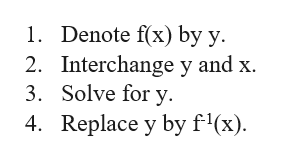Question
1 views

if f(x) = (3x + 2)/5 then f-1(x) =

check_circle

Step 1

We are given that f(x) = (3x + 2)/5. We need to find f-1(x).

For finding the i...help_outlineImage Transcriptionclose1. Denote f(x) by y 2. Interchange y and x 3. Solve for y 4 Replace y by f'(x) fullscreen

### Want to see the full answer?

See Solution

#### Want to see this answer and more?

Solutions are written by subject experts who are available 24/7. Questions are typically answered within 1 hour.*

See Solution
*Response times may vary by subject and question.
Tagged in

### Math